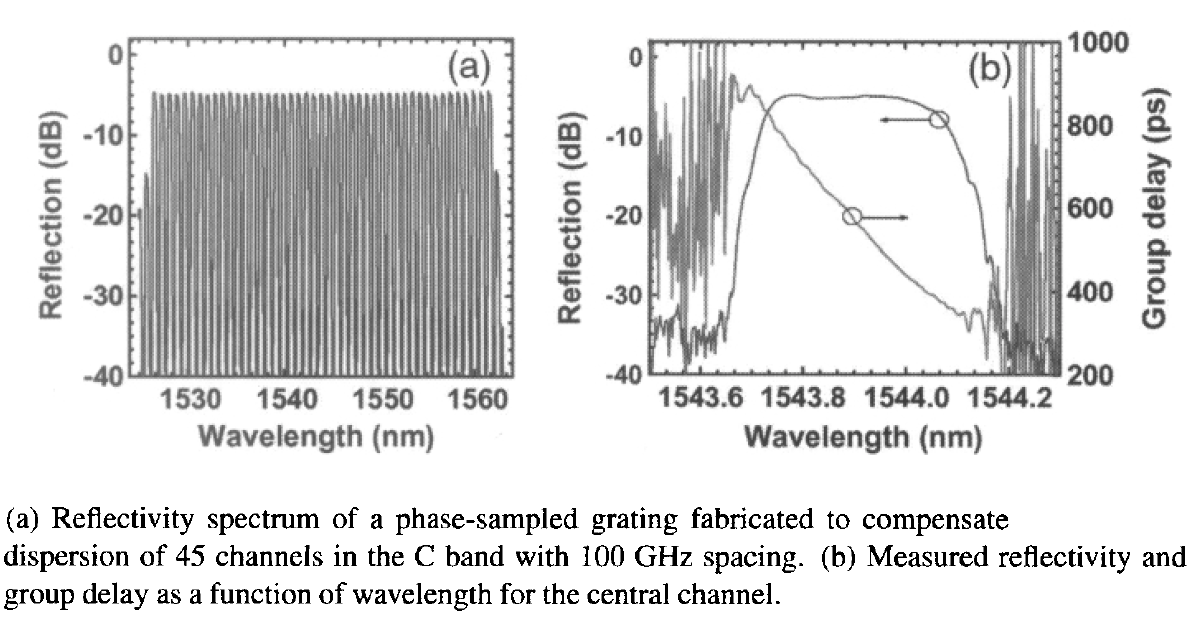# Dispersion Compensation with Fiber Bragg Gratings

The dispersion-compensating fibers (DCFs) discussed in the previous tutorial suffer from high insertion losses because of their relatively long lengths. They also enhance the impact of nonlinear effects within a long-haul system. Both of these issues can be resolved to a large extent by using fiber-based Bragg gratings for dispersion compensation.

In a fiber Bragg grating, the refractive index inside the core changes in a period fashion along the grating length. Because of this feature, the grating acts as an optical filter. More specifically, it develops a stop band in the form of a spectral region over which most of the incident light is reflected back. The stop band is centered at the Bragg wavelength λB that is related to the grating period Λ by the relation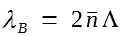, where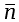is the average mode index. The periodic nature of index variations couples the forward- and backward-propagating waves at wavelengths close to the Bragg wavelength and, as a result, provides frequency-dependent reflectivity to the incident signal over a bandwidth determined by the grating strength. In essence, a fiber grating acts as a reflection filter. Although the use of such gratings for dispersion compensation was proposed in the 1980s, it was only during the 1990s that fabrication technology advanced enough that their use became practical.

#### 1. Constant-Period Gratings

In the simplest type of grating, the refractive index varies along the grating length in a periodic fashion as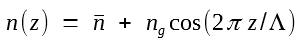where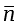is the average value of the refractive index and ng is the modulation depth. Typically, ng ~ 10-4 and Λ ~ 0.5 μm for fiber gratings designed to operate near the 1550-nm spectral region. Bragg gratings are analyzed using two coupled-mode equations that describe the coupling between the forward- and backward-propagating waves at a given frequency ω = 2πc/λ. These equations have the formwhere Af and Ab are the field amplitudes of the forward- and backward-propagating waves, respectively, andPhysically, δ represents detuning from the Bragg wavelength, κ is the coupling coefficient, and Γ is the confinement factor.

The coupled-mode equations can be solved analytically owing to their linear nature. Most of the input light is reflected when optical frequency is close to the Bragg wavelength. The transfer function of the grating, acting as a reflective filter, is found to be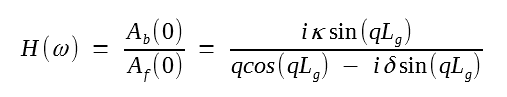where q2 =  δ2 - κ2 and Lg is the grating length. When incident wavelength falls in the region - κ < δ < κ, q becomes imaginary, and most of the light is reflected back by the grating (reflectivity becomes nearly 100% for κLg > 3). This region constitutes the stop band of the grating.

As discussed in other tutorials, the dispersion characteristics of the grating are related to the frequency dependence of the phase of H(ω). It is easy to show that the phase is nearly linear inside the stop band. Thus, grating-induced dispersion exists mostly outside the stop band, a region in which grating transmits most of the incident light. In this region (|δ| > κ), the dispersion parameters of a fiber grating are given bywhere vg is the group velocity.

Grating dispersion is anomalous () on the high-frequency or "blue" side of the stop band, where δ is positive and the carrier frequency exceeds the Bragg frequency. In contrast, dispersion becomes normal () on the low-frequency or "red" side of the stop band. The red side can be used for compensating the dispersion of standard fibers near 1.55 μm (β2 ≈ -21 ps2/km).  Sincecan exceed 1,000 ps2/cm, a single 2-cm-long grating can compensate dispersion accumulated over 100 km of fiber. However, higher-order dispersion of the grating, nonuniform transmission, and rapid variations of |H(ω)| close to stop-band edges make constant-period fiber gratings far from being practical for dispersion compensation.

An apodization technique is used in practice to improve the grating response. In an apodized grating, the index change ng is nonuniform across the grating, resulting in a z-dependent κ. Typically, as shown in figure (a) below, κ is uniform in the central region of length L0 and tapers down to zero at both ends over a short length Lt for a grating of length L ≡ L0 + 2Lt. Figure (b) below shows the measured reflectivity spectrum of an apodized 7.5-cm-long grating.

The reflectivity exceeds 90% within the stop band designed to be about 0.17-nm wide. The sharp drop in the reflectivity at both edges of the stop band is due to apodization.In another approach, the grating is fabricated such that κ varies linearly over its entire length. In a 1996 experiment, such an 11-cm-long grating was used to compensate the GVD acquired by a 10-Gb/s signal over 100 km of standard fiber. The coupling coefficient κ(z) varied smoothly from 0 to 6 cm-1 over the grating length. Such a grating compensated GVD over 106 km for a 10-Gb/s signal with only a 2-dB power penalty at a bit-error-rate  (BER) of 10-9. In the absence of the grating, the penalty was infinitely large because of the existence of a BER floor.

Tapering of the coupling coefficient along the grating length can also be used for dispersion compensation when the signal wavelength lies within the stop band, and the grating acts as a reflection filter. Numerical solutions of the coupled-mode equations for a uniform-period grating for which κ(z) varies linearly from 0 to 12 cm-1 over the 12-cm length show that such a grating exhibits a V-shaped group-delay profile, centered at the Bragg wavelength. It can be used for dispersion compensation if the wavelength of the incident signal is offset from the center of the stop band such that the signal spectrum sees a linear variation of the group delay. Such a 8.1-cm-long grating was capable of compensating the GVD acquired over 257 km of standard fiber by a 10-Gb/s signal.

Although uniform gratings have been used for dispersion compensation, they suffer from a relatively narrow stop band (typically <0.1 nm) and cannot be used at high bit rate. This shortcoming can be overcome by using chirped gratings, a topic we turn to next.

#### 2. Chirped Fiber Gratings

Chirped fiber gratings have a relatively broad stop band and were proposed for dispersion compensation as early as 1987. The optical period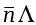in a chirped grating is not constant but changes over its length. Since the Bragg wavelength (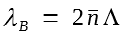) also varies along the grating length, different frequency components of an incident optical pulse are reflected at different points, depending on where the Bragg condition is satisfied locally. In essence, the stop band of a chirped fiber grating results from overlapping of many mini stop bands, each shifted as the Bragg wavelength shifts along the grating. The resulting stop band can be more than 10 nm wide, depending on the grating length. Such gratings can be fabricated using several different methods.

It is easy to understand the operation of a chirped fiber grating from the figure below, where the low-frequency components of a pulse are delayed more because of increasing optical period (and the Bragg wavelength).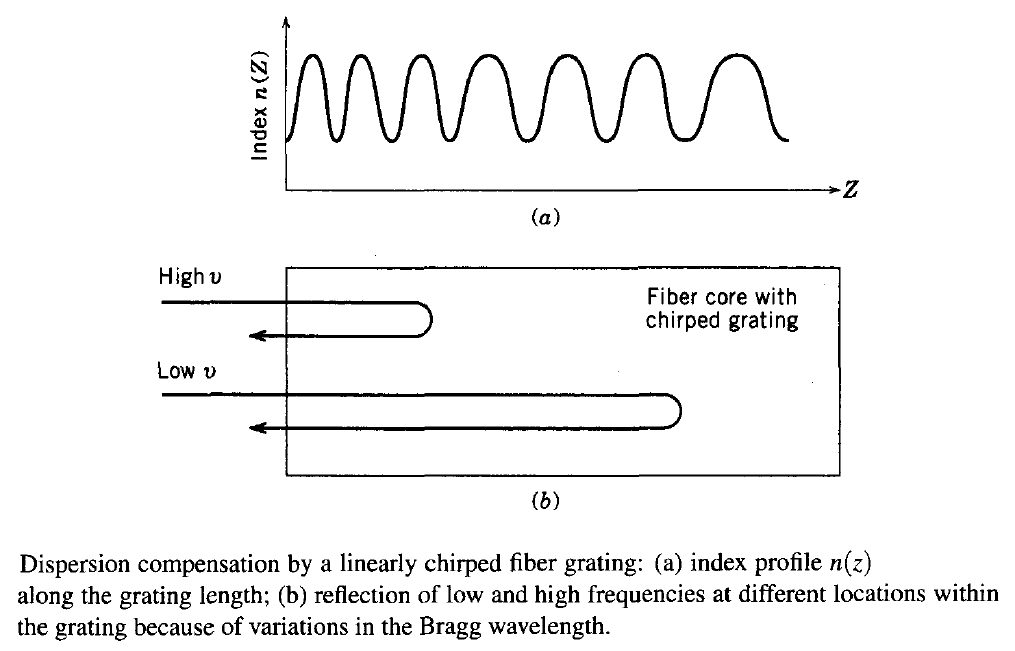This situation corresponds to anomalous GVD. The same grating can provide normal GVD if it is flipped (or if the light is incident from the right). Thus, the optical periodof the grating should decrease for it to provide normal GVD.

From this simple picture, the dispersion parameter Dg of a chirped grating of length Lg can be determined by using the relation TR = DgLgΔλ, where TR is the round-trip time inside the grating and Δλ is the difference in the Bragg wavelengths at the two ends of the grating. Since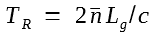the grating dispersion is given by a remarkably simple expression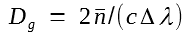As an example, Dg ≈ 5 x 107 ps/(km-nm) for a grating bandwidth Δλ = 0.2 nm.  Because of such large values of Dg, a 10-cm-long chirped grating can compensate for the GVD acquired over 300 km of standard fiber.

Chirped fiber gratings were employed for dispersion compensation during the 1990s in several transmission experiments. In a 10-Gb/s experiment, a 12-cm-long chirped grating was used to compensate dispersion accumulated over 270 km of fiber. Later, the transmission distance was increased to 400 km using a 10-cm-long apodized chirped fiber grating. This represents a remarkable performance by an optical filter that is only 10 cm long. When compared to DCFs, fiber gratings offer lower insertion losses and do not enhance the nonlinear degradation of the signal.

It is necessary to apodize chirped gratings to avoid group-delay ripples that affect system performance. Mathematically, index variations across the grating takes the following form for an apodized chirped grating: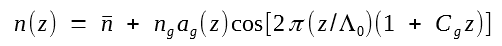where ag(z) is the apodization function, Λ0 is the value of the grating period at z = 0, and Cg is the rate at which this period changes with z. The apodization function is chosen such that ag = 0 at the two grating ends but becomes 1 in the central part of the grating. The fraction F of the grating length over which ag changes from 0 to 1 plays an important role.

The following figure shows the reflectivity and the group delay (related to the phase derivative dφ/dω) calculated as a function of wavelength by solving the coupled-mode equations for several 10-cm-long gratings with different values of peak reflectivities and the apodization function F. The chirp rate Cg = 6.1185 x 10-4 m-1 was constant in all cases. The modulation depth ng was chosen such that the grating bandwidth was wide enough to fit a 10-Gb/s signal within its stop band. Dispersion characteristics of such gratings can be further optimized by choosing the apodization profile ag(z) appropriately.It is apparent from the figure above that apodization reduces ripples in both the reflectivity and group-delay spectra. Since the group delay should vary with wavelength linearly to produce a constant GVD across the signal spectrum, it should be as ripple-free as possible. However, if the entire grating length is apodized (F = 1), the reflectivity ceases to be constant across the pulse spectrum, an undesirable situation. Also, reflectivity should be as large as possible to reduce insertion losses. In practice, gratings with 95% reflectivity and F = 0.7 provide the best compromise for 10-Gb/s systems.

The figure below shows the measured reflectivity and group delay spectra for a 10-cm-long grating whose bandwidth of 0.12 nm is chosen to ensure that a 10-Gb/s signal fits within its stop band. The slope of the group delay (about 5,000 ps/nm) is a measure of the dispersion-compensation capability of the grating. Such a grating can recover a 10-Gb/s signal by compensating the GVD acquired over 300 km of the standard fiber.From this expression:it is clear that Dg of a chirped grating is ultimately limited by the bandwidth Δλ over which GVD compensation is required, which in turn is determined by the bit rate B. This creates a dilemma because as the stop-band bandwidth is increased to accommodate a high-bit-rate signal, the grating dispersion Dg decreases. Cascading of two or more gratings can solve this problem to some extent. In a 1996 experiment, two chirped fiber gratings were cascaded to compensate fiber dispersion over 537 km. In another 1996 experiment, the transmission distance of a 10-Gb/s signal was extended to 700 km by using a 10-cm-long chirped grating in combination with a phase-alternating duobinary scheme.

A drawback of chirped fiber gratings is that they work as a reflection filter. A 3-dB fiber coupler can be used to separate the reflected signal from the incident one. However, its use imposes a 6-dB loss that adds to other insertion losses. An optical circulator reduces insertion losses to below 2 dB. Several other techniques can be used. For example, two or more fiber gratings can be combined to form a transmission filter that provides dispersion compensation with relatively low insertion losses. A single grating can be converted into a transmission filter by introducing a phase shift in the middle of the grating. A Moiré grating, constructed by superimposing two chirped gratings formed on the same piece of fiber, also has a transmission peak within its stop band. The bandwidth of such transmission filters is relatively small.

A major drawback of a fiber grating is that its transfer function exhibits a single peak. Plus, a single grating cannot compensate the dispersion of several WDM channels unless its design is modified. Several different approaches can be used to solve this problem. In one approach, chirped fiber gratings are fabricated with a wide stop band (>10 nm) by increasing their length. Such a grating can be used in a WDM system if the number of channels is small enough that the total signal bandwidth fits inside its stop band. In a 1999 experiment, a 6-nm-bandwidth chirped grating was used for a four-channel WDM system, each channel operating at 40 Gb/s. When the WDM-signal bandwidth is much larger than that, one can use several cascaded chirped gratings in series such that each grating reflects one channel and compensates its dispersion. The advantage of this technique is that the gratings can be tailored to match the dispersion experienced by each channel, resulting in automatic dispersion-slope compensation.

The following figure shows the cascaded-grating scheme schematically for a four-channel WDM system. Every 80 km, a set of four gratings compensates the GVD for all channels, while two optical amplifiers take care of all losses. By 2000, this approach was applied to a 32-channel WDM system with 18-nm bandwidth. Six chirped gratings, each with a 6-nm-wide stop band, were cascaded to compensate GVD for all channels simultaneously.#### 3. Sampled Gratings

The cascaded-gratings approach becomes cumbersome as the number of channels in a WDM system becomes large. A new kind of fiber grating, referred to as the sampled grating, has been developed to solve this problem. Such devices have double periodicity and are also known as superstructure gratings. Fiber-based sampled gratings were first made in 1994. Since then, they have attracted considerable attention for dispersion compensation.

A sampled grating consists of multiple subgratings separated from each other by a section of uniform index (each subgrating is a sample, hence the name "sampled" grating). The figure below shows a sampled grating schematically. In practice, such a structure can be realized by blocking certain regions through an amplitude mask during fabrication of a long grating such that κ = 0 in the blocked regions. In can also be made by etching away parts of an existing grating. In both cases, κ(z) varies periodically along z. It is this periodicity that that modifies the stop band of a uniform grating. If the average indexalso changes with the same period, both δ and κ become periodic in the coupled-mode equations. The solution of these equations shows that a sampled grating has multiple stop bands separated from each other by a constant amount. The frequency spacing Δνp among neighboring reflectivity peaks is set by the sample period Λs as Δνp = c/(2ngΛs) and is controllable during the fabrication process. Moreover, if each subgrating is chirped, the dispersion characteristics of each reflectivity peak are governed by the amount of chirp introduced.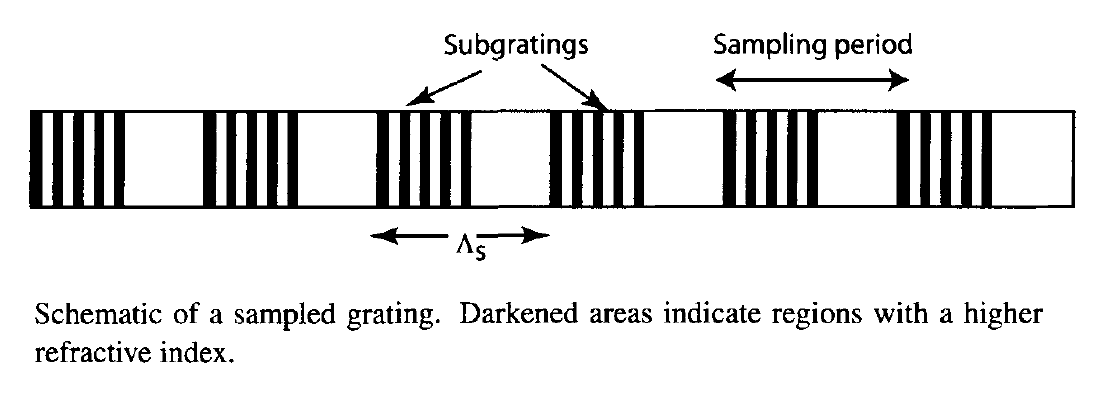A sampled grating is characterized by a periodic sampling function S(z). The sampling period Λs of about 1 mm is chosen so that Δνp is close to 100 GHz (typical channel spacing for WDM systems). In the simplest kind of grating, the sampling function is a "rect" function such that S(z) = 1 over a section of length fsΛs and 0 over the remaining portion of length (1 - fss. However, this is not the optimum choice because it leads to a transfer function in which each peak is accompanied by multiple subpeaks. The reason is related to the fact that the shape of the reflectivity spectrum is governed by the Fourier transform of S(z). This can be seen by multiplying ng in the constant-period gratings index expression:with S(z) and expanding S(z) in a Fourier series to obtainwhere Fm is the Fourier coefficient, β0 = π/Λ0 is the Bragg wave number, and βs is related to the sampling period Λs as βs = π/Λs. In essence, a sampled grating behaves as a collection of multiple gratings whose stop bands are centered at wavelengths λm = 2π/βm, where βm = β0 + mβs, and m is an integer. The peak reflectivity associated with different stop bands is governed by the Fourier coefficient Fm.

A multipeak transfer function, with nearly constant reflectivity for all peaks, can be realized by adopting a sampling function of the form S(z) = sin(az)/az, where a is a constant. Such a "sinc" shape function was used in 1998 to fabricate 10-cm-long gratings with up to 16 reflectivity peaks separated by 100 GHz. A 1999 experiment used such a sampled grating for a four-channel WDM system.

As the number of channels increases, it becomes more and more difficult to compensate the GVD of all channels at the same time because such a grating does not compensate for the dispersion slope of the fiber. This problem can be solved by introducing a chirp in the sampling period Λs, in addition to the chirping of the grating period Λ. In practice, a linear chirp is used. The amount of chirp depends on the dispersion slope of the fiber as δΛss = |S/D|Δλch, where Δλch is the channel bandwidth and δΛs is the change in the sampling period over the entire grating length. The figure below shows the reflection and dispersion characteristics of a 10-cm-long sampled grating designed for 8 WDM channels with 100-GHz spacing. For this grating, each subgrating was 0.12 mm long and the 1-mm sampling period was changed by only 1.5% over the 10-cm grating length.The preceding approach becomes impractical as the number N of WDM channels increases because it requires a large index modulation (ng grows linearly with N). A solution is offered by sampled gratings in which the sampling function S(z) modifies the phase of κ, rather than changing its amplitude; the modulation depth in this case grows only as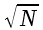. The phase-sampling technique has been used with success for making tunable semiconductor lasers. Recently, it has been applied to fiber gratings. In contrast with the case of amplitude sampling, index modulations exist over the entire grating length. However, the phase of modulation changes in a periodic fashion with a period Λs that itself is chirped along the grating length. Mathematically, index variations can be written in the form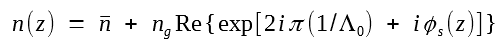where ng is the constant modulation amplitude, Λ0 is the average grating period, and the phase φs(z) varies in a periodic fashion. By expanding exp(iφs) in a Fourier series, n(z) can be written in the form ofwhere Fm depends on how the phase φs(z) varies in each sampling period. The shape of the reflectivity spectrum and dispersion characteristics of the grating can be tailored by controlling Fm and by varying the magnitude of the chirp in the grating and sampling periods.

As an example, figure (a) below shows the measured reflectivity as a function of wavelength for a phase-sampled grating fabricated to compensate dispersion of 45 channels with 100 GHz spacing within the C band. Figure (b) below shows wavelength dependence of the group delay for the central channel located near 1543.9 nm. The magnitude of dispersion estimated from the slope of group delay is about -1374 ps/nm. Such a grating can compensate simultaneously dispersion acquired over 80 km of standard fiber for 45-channel WDM system. A phase mask was used to make this phase-sampled grating. The same technique was used to make an 81-channel grating. With the simultaneous use of two phase-sampling functions, such gratings can be designed to cover the S, C, and L bands.